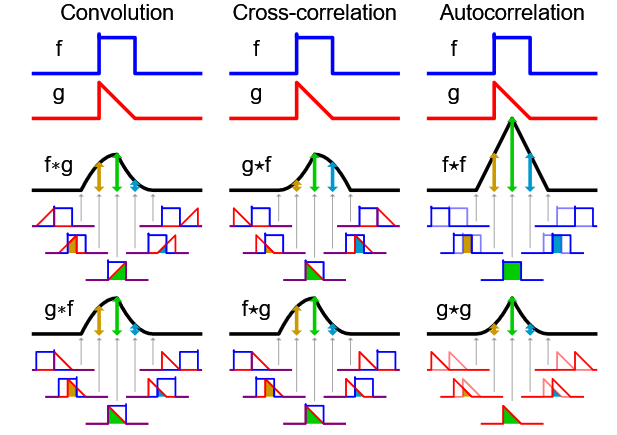# Convolution & Cross-correlation

The primary implement of convolution and cross-correlation.

# Notes

You can refer to the complete notes from 04 Notes

## Linear Systems(Filter)

Denotes the input function:

$$f[m,n]$$

Filter is used to convert input function to an output(or response) function:

$$g[m,n]$$

$\mathcal{S}$ is refered to as system operator which maps a member of the set of possible

outputs $g[m,n]$ to a member of the set of possible inputs $f[m,n]$. When using notation involving $\mathcal{S}$, we can write that

\begin{aligned} \mathcal{S}[g]&=f \\ \mathcal{S}\left\{f[m,n]\right\}&=g[m,n] \\ f[m,n]&\mapsto{g[m,n]} \end{aligned}

### Examples of Filters

#### Moving Average

If we want to smooth or blur the image, we can set the value of a pixel to be the average of its neighboring pixels:

$$g[m,n]=\frac{1}{9}\sum_{i=-1}^{1}\sum_{j=-1}^1f[m-i,n-j]$$

#### Image Segmentation

The value of a pixel can be set:

$$g[m,n]=\begin{cases} 255 & f[m,n]\geq{t}\\ 0&\text{otherwise} \end{cases}$$

### Properties of Systems

Not all systems will have the following(or any) of these properties in general. You can refer to the notes or slide about other properties.

#### Amplitude Properties

1. Superposition(叠加原理)

$$\mathcal{S}[\alpha{f_i[n,m]}+\beta{f_j[n,m]]}=\alpha\mathcal{S}[f_i[n,m]]+\beta\mathcal{S}[f_j[n,m]]$$

#### Properties of Systems

1. Shift Invariance(平移不变性)

$$f[n-m_0,n-n_0]\xrightarrow{\mathcal{S}}g[m-m_0,n-n_0]$$

### Linear Systems

A linear system is a system that satisfies the property of superposition.

Linear systems are also known as impulse response(脉冲响应) of a system $\mathcal{S}$. Consider a function $\delta_2[m,n]$:

$$\delta_2[m,n]=\begin{cases} 1&m=0\text{ and }n=0\\ 0&\text{otherwise} \end{cases}$$

The response $r$:

$$r=\mathcal{S}[\delta_2]$$

A simple linear shift-invariant system is a system that shifts the pixels of an image, based on the shifting property of the delta function.

$$f[m,n]=\sum_{i=-\infty}^\infty\sum_{j=-\infty}^\infty{f[i,j]\delta_2[m-i,n-j]}$$

Then we can use the superposition property to write any linear shift-invariant system as a weighted sum of such shifting system:

$$\alpha_1\sum_{i=-\infty}^\infty\sum_{j=-\infty}^\infty{f[i,j]\delta_{2,1}[m-i,n-j]}+\alpha_2\sum_{i=-\infty}^\infty\sum_{j=-\infty}^\infty{f[i,j]\delta_{2,}[m-i,n-j]}+\cdots$$

We can define the filter $h$ of a linear shift-invariant system as

$$h[m,n]=\alpha_1\delta_{2,1}[m-i,n-j]+\alpha_2\delta_{2,2}[m-i,n-j]+\cdots$$

Linear shift invariant systems(LSI):

• Systems that satisfy the superposition property
• Have an impulse response: $\mathcal{S}[\delta_2[n,m]]=\delta_2[n,m]$
• Discrete convolution: $f[n,m]*h[n,m]$(multiplication of shifted-version of impulse response by original function)

# Convolution and Correlation

## Convolution

The impulse function $\delta[n]$ is defined to be 1 at $n=1$ and 0 elsewhere. For an arbitrary input signal $x$ can be written as $x[n]=\sum_{k=-\infty}^\infty{x[k]\delta[n-k]}$. If we pass the equation into a linear, shift-invariant system, the output is $y[n]=\sum_{k=-\infty}^\infty{x[k]h[n-k]}$, i.e the convolution of the signal $x$ with the impulse response $h$.

However, an image is written in form of matrix. For a linear, shift-invariant system, the output is $y[n,m]=\sum_{i=-\infty}^\infty\sum_{j=-\infty}^\infty{x[i,j]h[n-i,m-j]}$

,i.e the convolution of the signal $x$ with the impulse response $h$ in 2 dimensions.

## Correlation(互相关)

Cross Correlation is often used to measure the similarity of two images. cross correlation is the same as convolution, except that the filter kernel is not flipped. Two-dimensional cross correlation is:

$$r[k,l]=\sum_{m=-\infty}^\infty\sum_{n=-\infty}^\infty{f[m+k,n+l]}g[m,n]$$

## Summary

The steps for discrete convolution are:

• Fold $h[k,l]$(折叠) about origin to form $h[-k]$
• Shift th folded results by $n$ to form $h[n-k]$
• Multiply $h[n-k]$ by $f[k]$
• Sum over all $k$
• Repeat for every n

However, cross correlation doesn't have to fold the filter. The convolution amis to calculate the value of weighted $f[m,n]$ by folded and shifted filter $f[k,l]$In this picture, we assume function $f$ and its height is 1.0. The value of the result at 5 different points is indicated by the shaded area below each point. Also, the symmetry of f is the reason f*g and $f\star{g}$ are identical in this example.

Cross correlation doesn't satisfy commutative property(交换律):

$$f\star{g}(t)=(g\star{f})(-t)$$

However, convolution satisfy commutative property:

$$f*g=g*f$$

# How to run

2. hw1.ipynb is interface for user to debug/visualize the code in filter.py